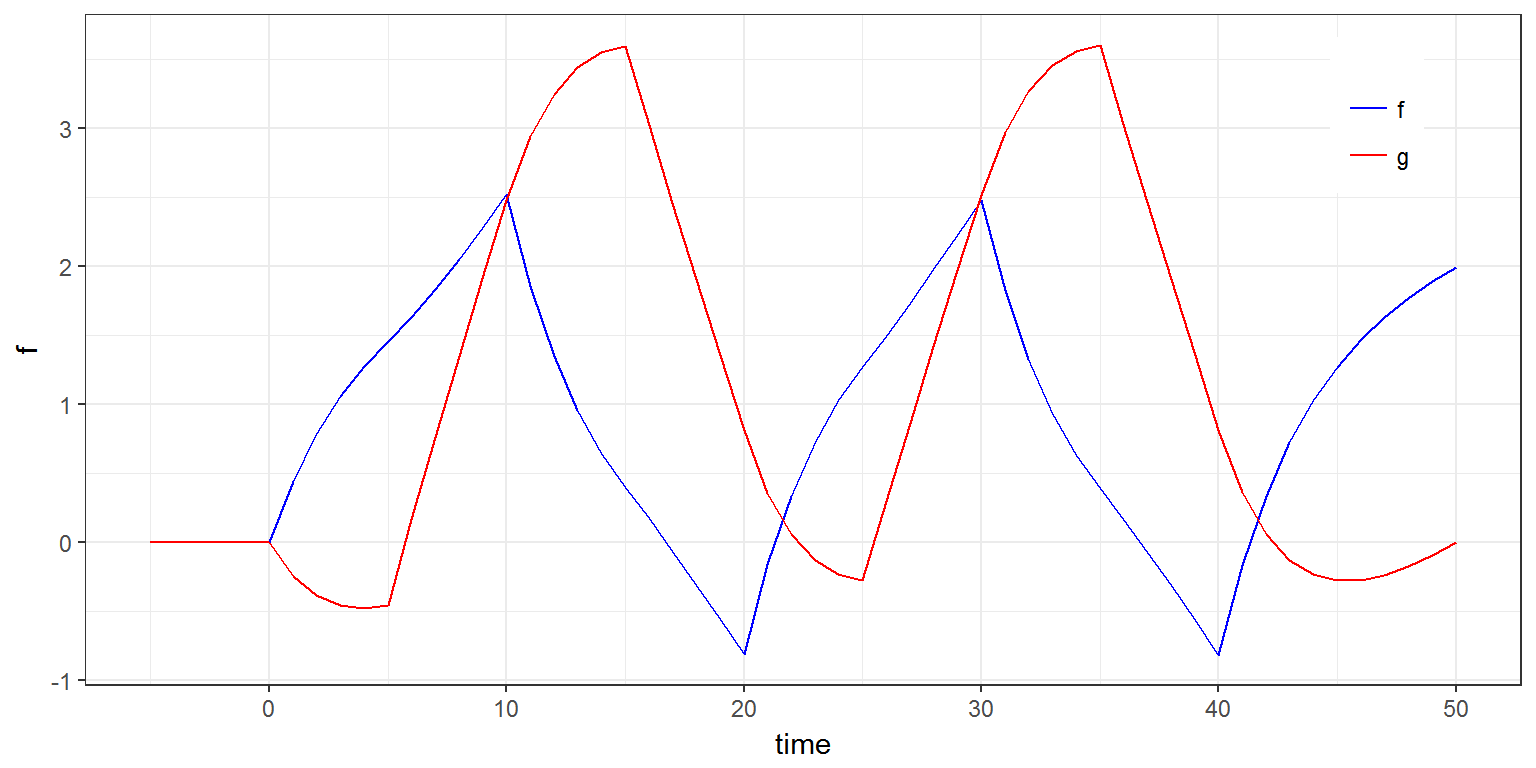R script: regression.R

$$\newcommand{\esp}{\mathbb{E}\left(#1\right)} \newcommand{\var}{\mbox{Var}\left(#1\right)} \newcommand{\deriv}{\dot{#1}(t)} \newcommand{\prob}{ \mathbb{P}\!(#1)} \newcommand{\eqdef}{\mathop{=}\limits^{\mathrm{def}}} \newcommand{\by}{\boldsymbol{y}} \newcommand{\bc}{\boldsymbol{c}} \newcommand{\bpsi}{\boldsymbol{\psi}} \def\pmacro{\texttt{p}} \def\like{{\cal L}} \def\llike{{\cal LL}} \def\logit{{\rm logit}} \def\probit{{\rm probit}} \def\one{{\rm 1\!I}} \def\iid{\mathop{\sim}_{\rm i.i.d.}} \def\simh0{\mathop{\sim}_{H_0}} \def\df{\texttt{df}} \def\res{e} \def\xomega{x} \newcommand{\argmin}{{\rm arg}\min_{#1}} \newcommand{\argmax}{{\rm arg}\max_{#1}} \newcommand{\Rset}{\mbox{\mathbb{R}}} \def\param{\theta} \def\setparam{\Theta} \def\xnew{x_{\rm new}} \def\fnew{f_{\rm new}} \def\ynew{y_{\rm new}} \def\nnew{n_{\rm new}} \def\enew{e_{\rm new}} \def\Xnew{X_{\rm new}} \def\hfnew{\widehat{\fnew}} \def\degree{m} \def\nbeta{d} \newcommand{\limite}{\mathop{\longrightarrow}\limits_{#1}} \def\ka{k{a}} \def\ska{k{\scriptscriptstyle a}} \def\kel{k{e}} \def\skel{k{\scriptscriptstyle e}} \def\cl{C{\small l}} \def\Tlag{T\hspace{-0.1em}{lag}} \def\sTlag{T\hspace{-0.07em}{\scriptscriptstyle lag}} \def\Tk{T\hspace{-0.1em}{k0}} \def\sTk{T\hspace{-0.07em}{\scriptscriptstyle k0}} \def\thalf{t{1/2}} \newcommand{\Dphi}{\partial_\pphi #1} \def\asigma{a} \def\pphi{\psi} \newcommand{\stheta}{{\theta^\star}} \newcommand{\htheta}{{\widehat{\theta}}}$$

# 1 Introduction

A regression variable is a variable $$x$$ which is a given function of time, which is not defined in the model but which is used in the model.

A regression variable is defined in the R script as a vector $$(x_1, x_2, \ldots, x_m)$$ together with a vector of times $$(t_1, t_2, \ldots, t_m)$$, where $$x_j=x(t_j)$$ is the value of $$x$$ at time $$t_j$$.
Then, this regression variable is used as an input of the Mlxtran code.

$$x$$ is only defined at time points $$t_1, t_2, \ldots, t_m$$ but $$x$$ is a function of time that should be defined for any $$t$$. Then, Mlxtran defines the function $$x$$ by intepolating the given values $$(x_1, x_2, \ldots, x_m)$$. In the current version of Mlxtran, interpolation is performed by using the last given value:

$x(t) = x_j \quad \text{for} \ \ t_j \leq t < t_{j+1}$

# 2 Examples

## 2.1 Example 1

Consider a Emax model where the effect $$E(t)$$ at time $$t$$ is function of the concentration $$C(t)$$: $E(t) = E_{\rm max} \frac{C(t)}{EC_{50} + C(t)}$ Assume that $$C$$ is given at times $$t_1, t_2, \ldots , t_m$$. We therefore use $$C$$ as a regression variable in the Mlxtran code model/regression1a.txt

[LONGITUDINAL]
input = {Emax, EC50, C}
C = {use=regressor}

EQUATION:
E = Emax*C/(C+EC50)


A regression variable is defined as an input argument regression of simulx. It is a list with three elements: name, time and value.

Assume in this example that $x(t) = e^{-0.1\, t}$ is given for $$t=0,1,2,\ldots,50$$.

t   <- seq(0,50,by=1)
reg <- list(name='C',
time=t,
value=exp(-0.1*t))

out <- list(name='E',
time=t)

res <- simulx( model     = "model/regression1a.txt",
parameter = c(Emax=100, EC50=0.3),
regressor = reg,
output    = out)

plot(ggplot(data=res$E) + geom_line(aes(x=time, y=E)))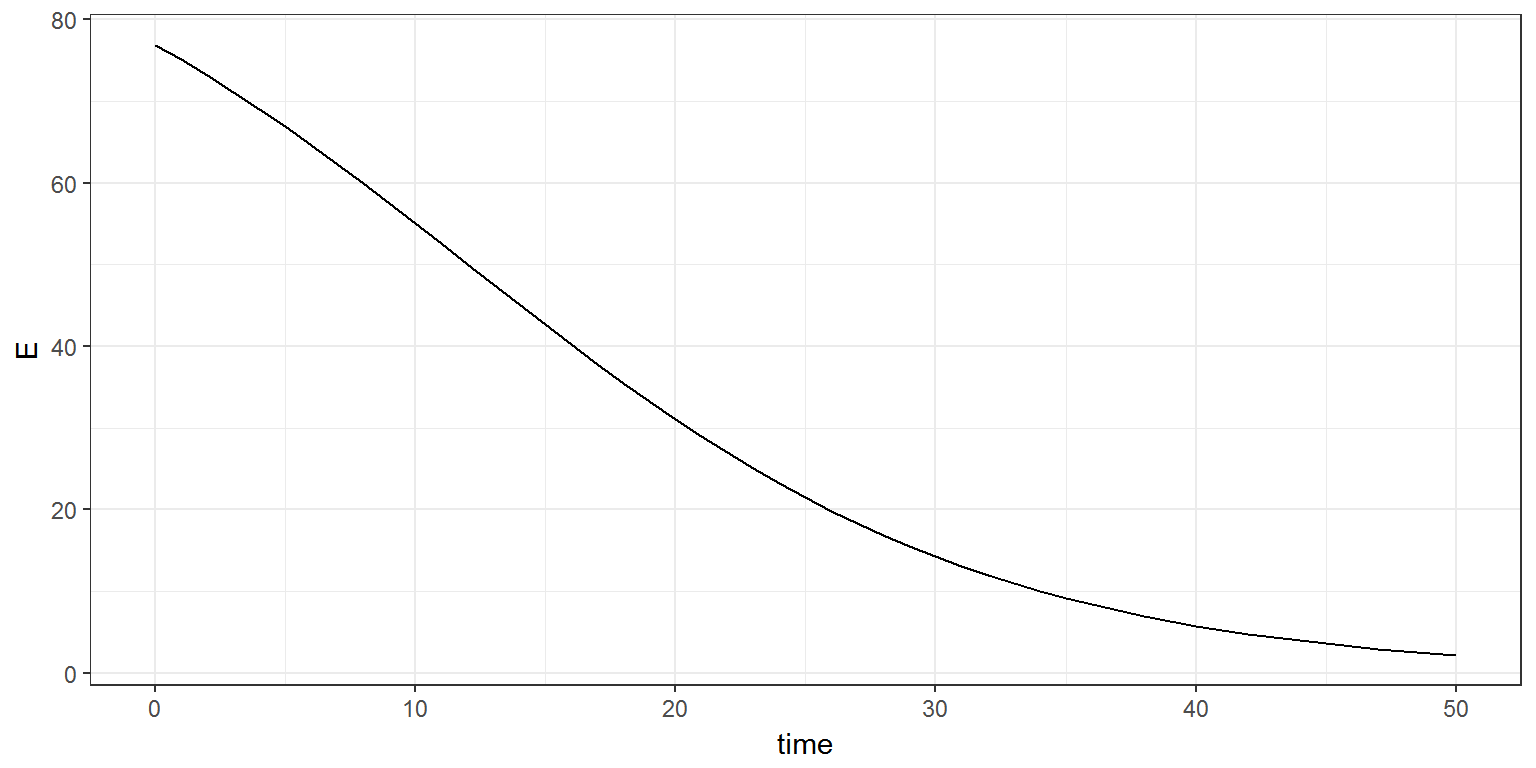If we want to define the regression variable as an output of the model, then, we have to define a new variable which will be defined as an output of the model: [LONGITUDINAL] input = {Emax, EC50, C} C = {use=regressor} EQUATION: E = Emax*C/(C+EC50) Cout = C  library(gridExtra) out <- list(name=c('E','Cout'), time=t) res <- simulx( model = "model/regression1b.txt", parameter = c(Emax=100, EC50=0.3), regressor = reg, output = out) names(res) <- "C" names(res$C)  <- c("time","C")
plot1 <- ggplot(data=res$C) + geom_line(aes(x=time, y=C)) plot2 <- ggplot(data=res$E) + geom_line(aes(x=time, y=E))
grid.arrange(plot1, plot2, ncol=2)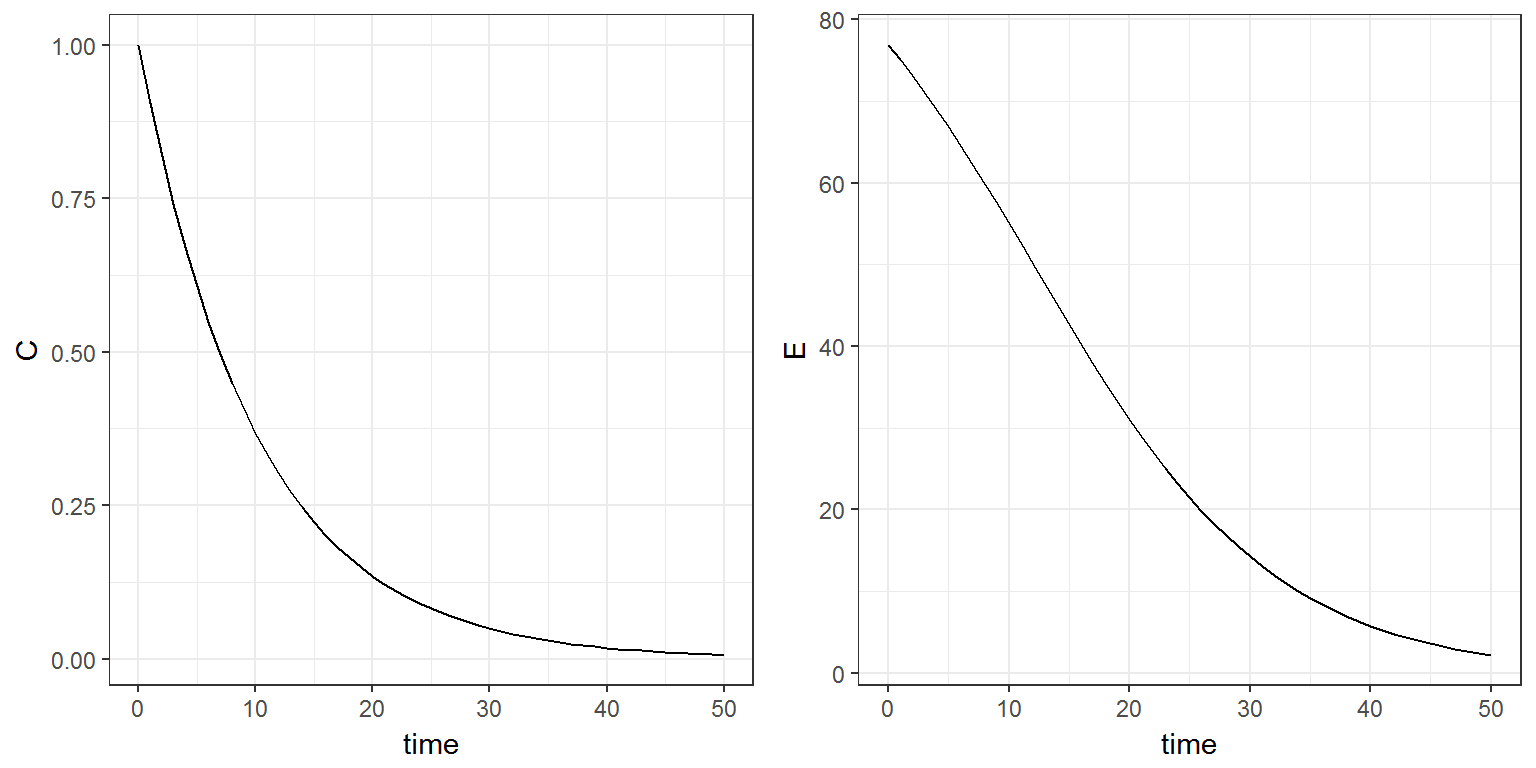## 2.2 Example 2

A regression variable can also be used as a state. Model regression2.txt assumes that there exist two states $$-1$$ and $$+1$$, such that the derivative of a variable $$f$$ depends on the state (here, $$\deriv{f}=a$$ if $$x(t)=1$$ and $$\deriv{f}=b$$ if $$x(t)=-1$$):

[LONGITUDINAL]
input = {a, b, x}
x = {use=regressor}

EQUATION:
if x==1
df = a
else
df = b
end
t0  = 0
f_0 = 0
ddt_f = df


In this example, the state changes every 5 hours: function $$f$$ is therefore a piecewise linear function, which slope abruptly changes every 5 hours.

x <- list(name='x',
time=c(0,5,10,20,25,30,40),
value=c(1,-1,1,-1,1,-1,1))

f <- list(name='f',
time=seq(0, 50, by=1))

res <- simulx( model     = "model/regression2.txt",
parameter = c(a=1, b=-0.5),
regressor = x,
output    = f)

print(ggplot(data=res$f) + geom_line(aes(x=time, y=f)))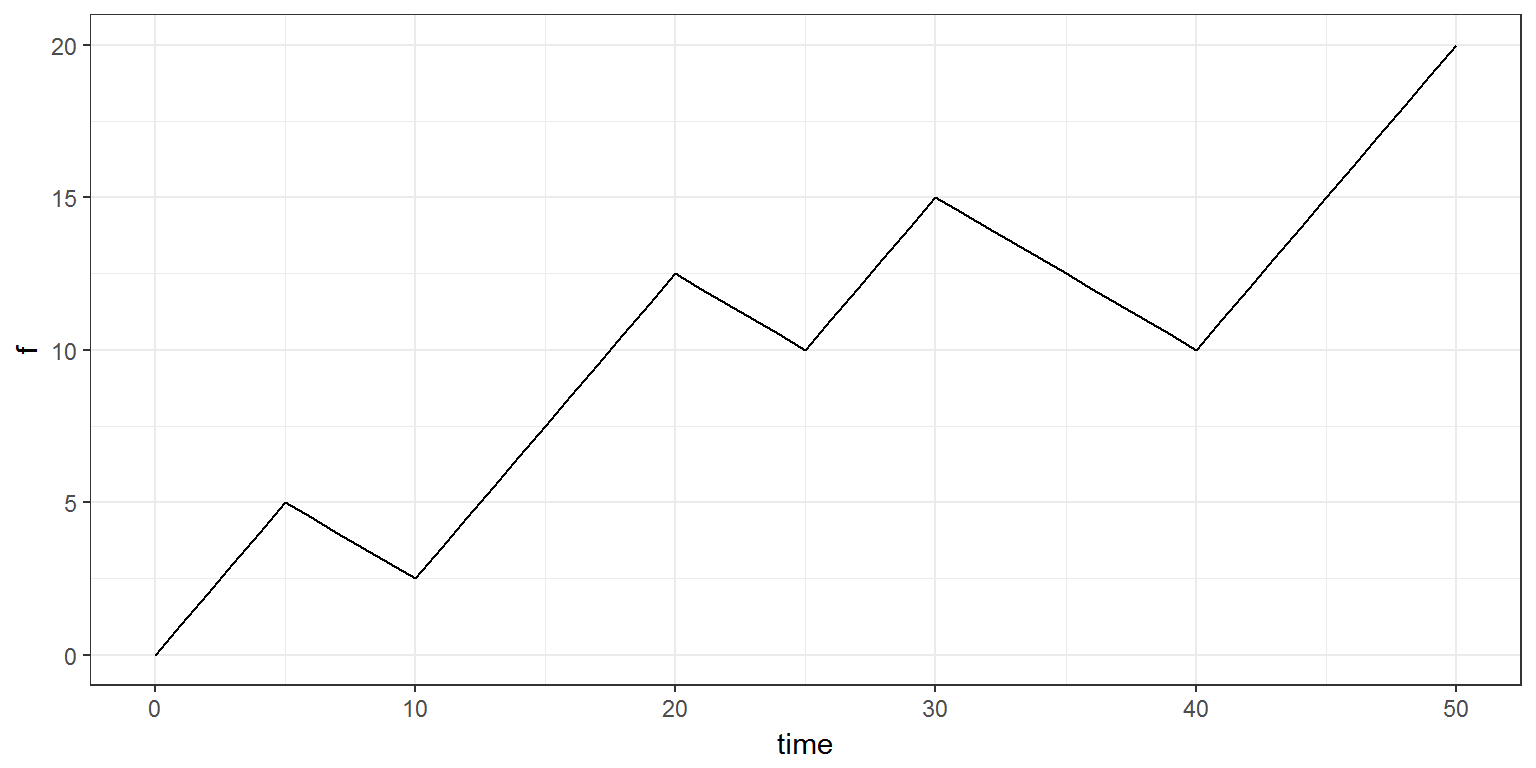## 2.3 Example 3 In this new model regression3a.txt, $$x$$ is used as rate function. [LONGITUDINAL] input = {k, f0, x} x = {use=regressor} EQUATION: t0 = 0 f_0 = f0 ddt_f = -k*f + x  This rate function is a piecewise constant function which changes abruptly every 5 hours. x <- list(name='x', time=c(0,5,10,20,25,30,40), value=c(1,-1,1,-1,1,-1,1)) f <- list(name='f', time=seq(-5, 50, by=1)) res <- simulx( model = "model/regression3a.txt", parameter = c(k=0.2, f0=0), regressor = x, output = f) print(ggplot(data=res$f) + geom_line(aes(x=time, y=f)))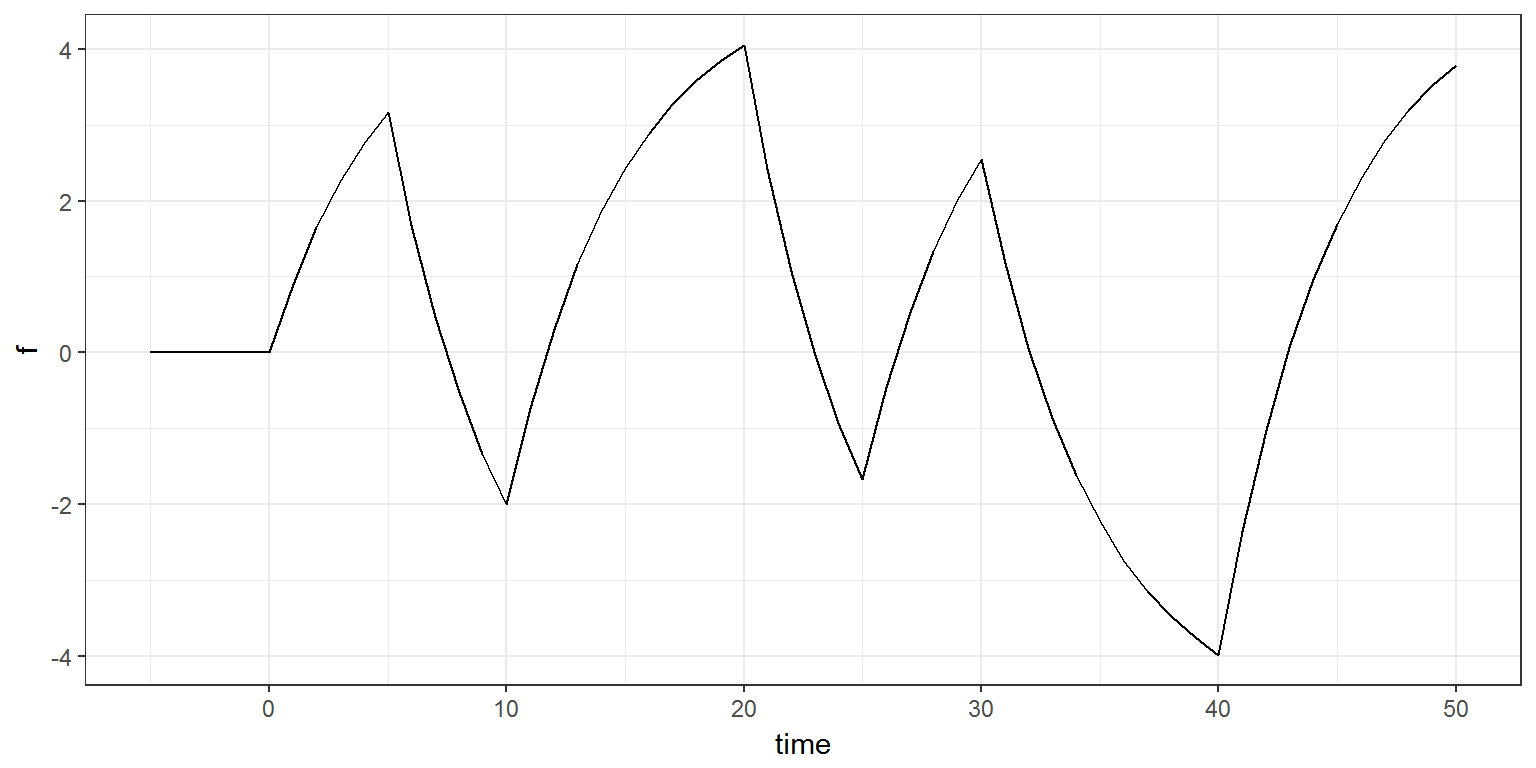Different values of the same regression variable can be defined per group. In this example, the regression variable $$x$$ is defined every at different time points for groups 1 and 2 (see Defining groups: part I for more details about the use of groups with ):

x1 <- list(name='x',
time=c(0,5,10,20,25,30,40),
value=c(1,-1,1,-1,1,-1,1))
x2 <- list(name='x',
time=c(0,4,14,24,34),
value=c(1,-0.5,1.5,-1,0.2))
g1  <- list(regressor = x1)
g2  <- list(regressor = x2)

f <- list(name='f',
time=seq(-5, 50, by=1))

res <- simulx( model     = "model/regression3a.txt",
parameter = c(k=0.2, f0=0),
group     = list(g1,g2),
output    = f)

print(ggplot(data=res$f) + geom_line(aes(x=time, y=f, colour=id)) + theme(legend.position=c(0.9, 0.85)))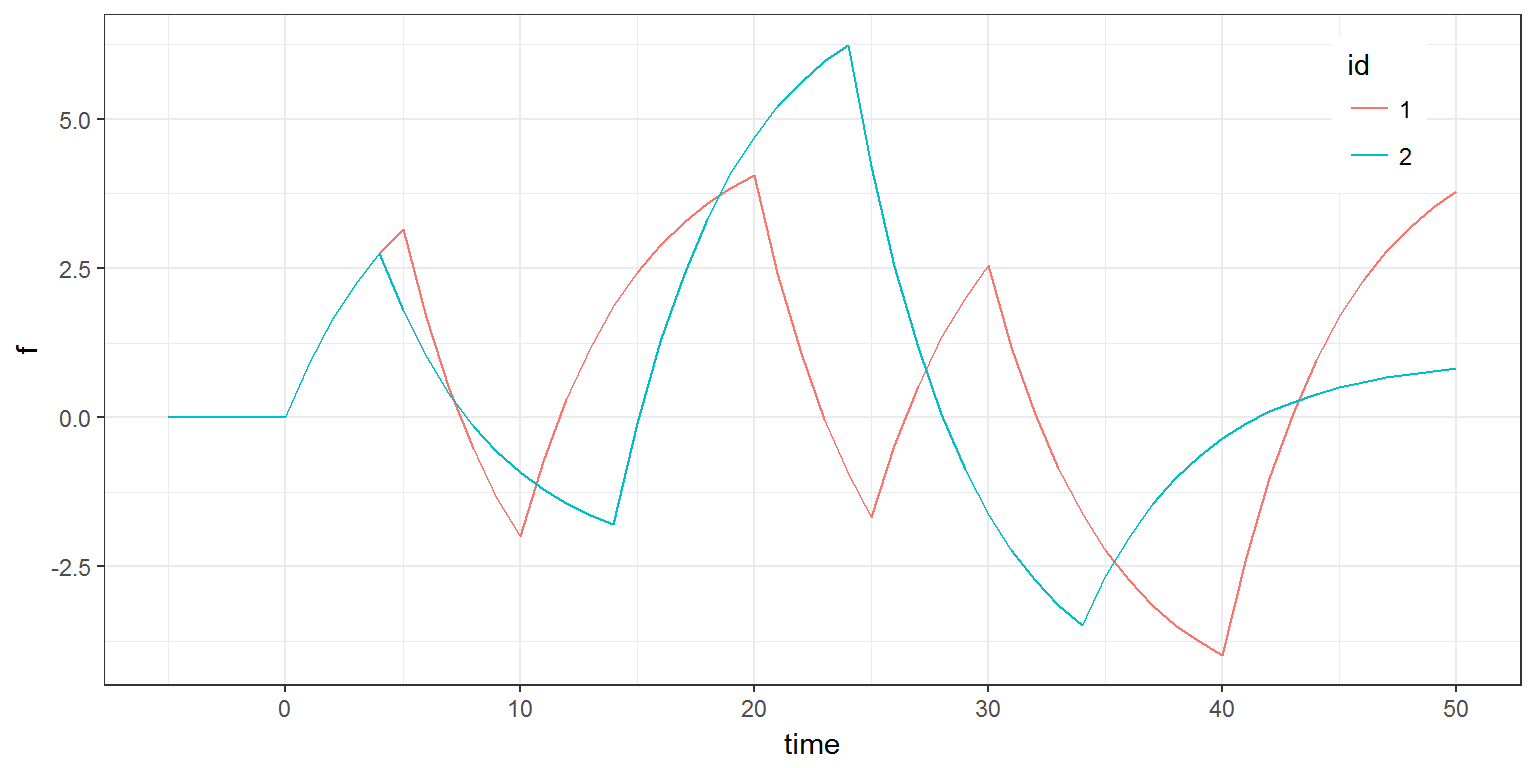Alternatively, individual values of the same regression variables can be defined in a datafile (converted here into a data frame). See Using data files and data frames for more details. x <- inlineDataFrame(" id time x 1 0 1 1 5 -1 1 10 1 1 20 -1 1 25 1 1 30 -1 1 40 1 2 0 1.0 2 4 -0.5 2 14 1.5 2 24 -1.0 2 34 0.2 ") f <- list(name='f', time=seq(-5, 50, by=1)) res <- simulx( model = "model/regression3a.txt", parameter = c(k=0.2, f0=0), regressor = x, output = f) print(ggplot(data=res$f) + geom_line(aes(x=time, y=f, colour=id)) + theme(legend.position=c(0.9, 0.85)))A regression variable is used to define a function of time $$f$$. This function computed at some given time points $$t_1, t_2, \ldots, t_n$$ can then be used as a prediction for some continuous data $$y_1, y_2, \ldots, y_n$$, as in the following model regression3b.txt:

[LONGITUDINAL]
input = {k, f0, a, x}
x = {use=regressor}

EQUATION:
t0  = 0
f_0 = f0
ddt_f = -k*f + x
DEFINITION:
y = {distribution=normal, prediction=f, sd=a}


The regression variable $$x$$, the function $$f$$ and the longitudinal data $$y$$ can be defined at different times (see Continuous data for more details about the definition of continuous data model).

x <- list(name='x',
time=c(0,5,10,20,25,30,40),
value=c(1,-1,1,-1,1,-1,1))

y <- list(name='y', time=seq(4, 48, by=1))

res <- simulx( model     = "model/regression3b.txt",
parameter = c(k=0.2, f0=0, a=0.5),
regressor = x,
output    = list(f,y))

print(ggplot() + geom_line(data=res$f, aes(x=time, y=f), colour="black") + geom_point(data=res$y, aes(x=time, y=y), colour="red"))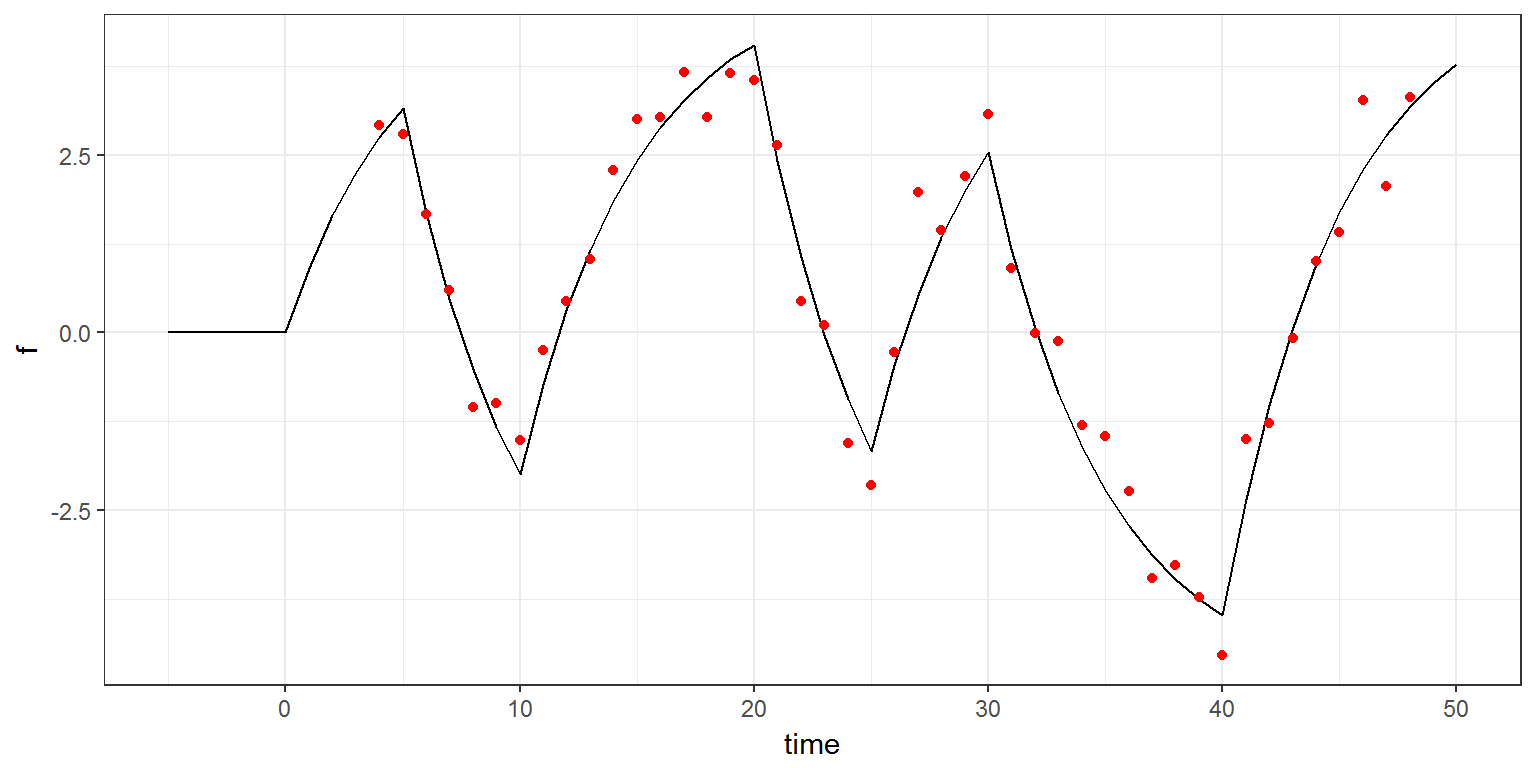## 2.4 Example 4

Several regression variables can be used in the same model (regression4.txt).

[LONGITUDINAL]
input = {k1, k2, f0, g0, x1, x2}
x1 = {use=regressor}
x2 = {use=regressor}

EQUATION:
t0  = 0
f_0 = f0
g_0 = g0
ddt_f = -k1*f + k2*g + x1
ddt_g =  k1*f - k2*g + x2


These regression variables can be defined at different time points. Each of them will be interpolated using the same method (i.e. using the last given value).

x1 <- list(name='x1',
time=c(0,10,20,30,40),
value=c(1,-1,1,-1,1)*0.5)
x2 <- list(name='x2',
time=c(5,15,25,35),
value=c(1,-1,1,-1)*0.3)

fg <- list(name=c('f','g'),
time=seq(-5, 50, by=1))

res <- simulx( model     = "model/regression4.txt",
parameter = c(k1=0.2, k2=0.1, f0=0, g0=0),
regressor = list(x1, x2),
output    = fg)

print(ggplot() + geom_line(data=res$f, aes(x=time, y=f, colour="blue")) + geom_line(data=res$g, aes(x=time, y=g, colour="red")) +
scale_colour_manual(name="",values=c('blue'='blue','red'='red'),labels=c('f','g')) +
theme(legend.position=c(0.9, 0.85)))